Anzeige

# EMPIRICAL APPLICATION OF SIMULATED ANNEALING USING OBJECT-ORIENTED METRICS TO INCREASE THE ACCURACY IN SOFTWARE EFFORT ESTIMATION

7. Jan 2016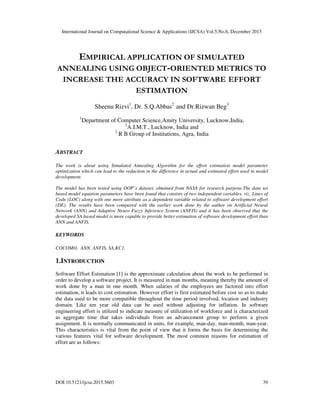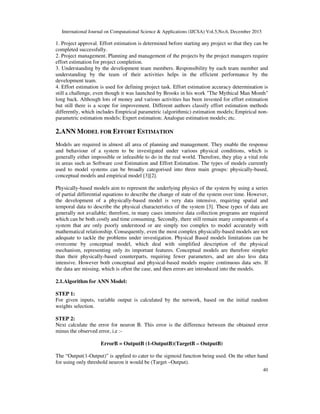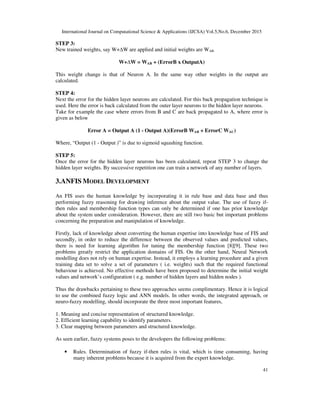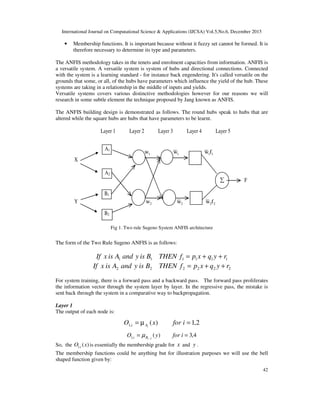Anzeige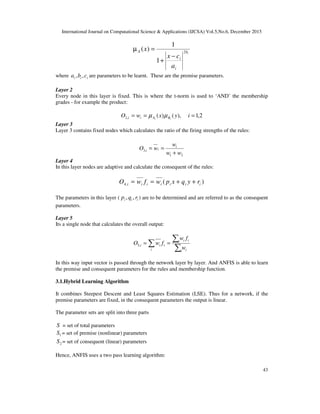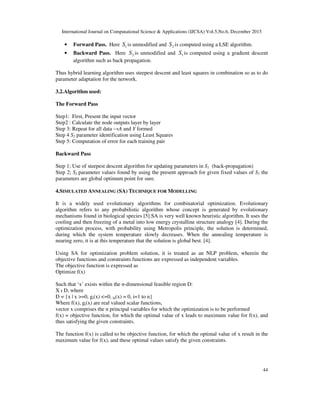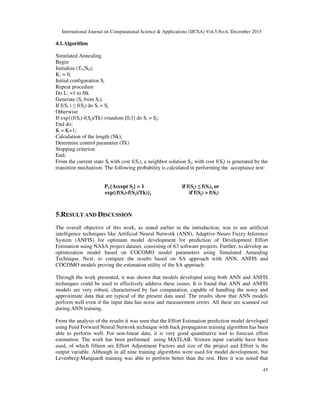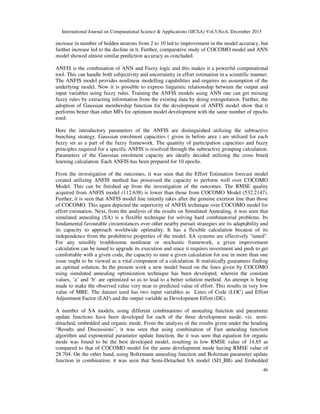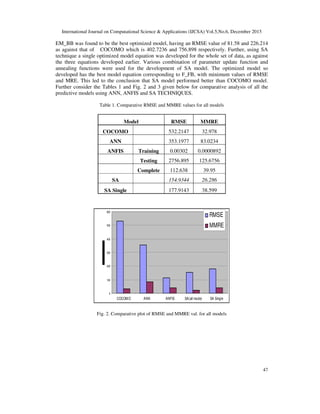Anzeige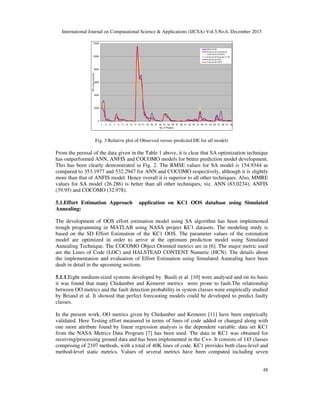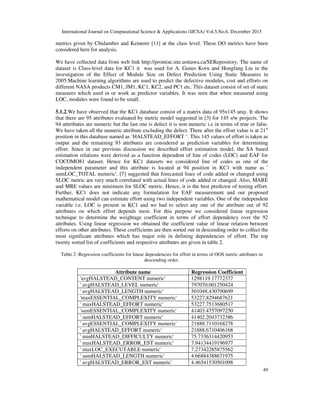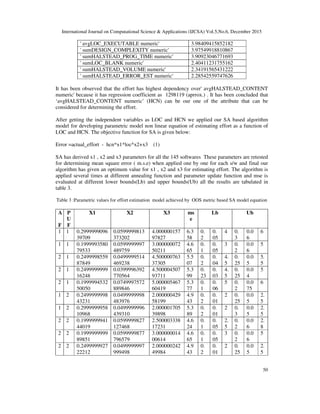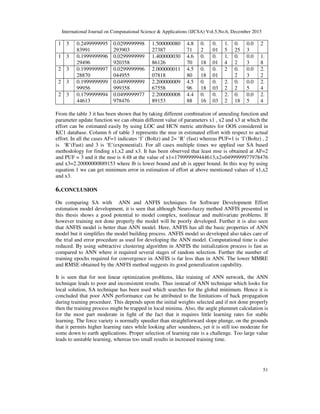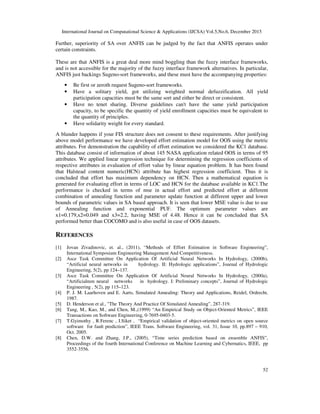Anzeige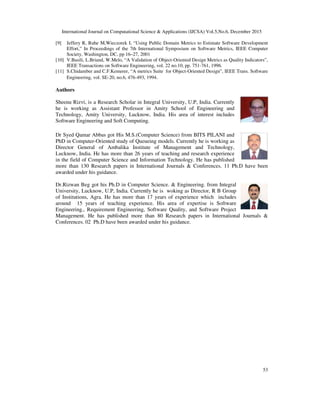Nächste SlideShare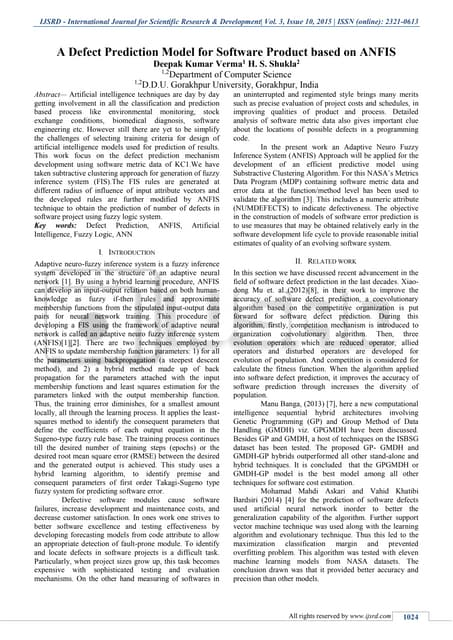A Defect Prediction Model for Software Product based on ANFIS
1 von 15
Anzeige

### EMPIRICAL APPLICATION OF SIMULATED ANNEALING USING OBJECT-ORIENTED METRICS TO INCREASE THE ACCURACY IN SOFTWARE EFFORT ESTIMATION

1. International Journal on Computational Science & Applications (IJCSA) Vol.5,No.6, December 2015 DOI:10.5121/ijcsa.2015.5603 39 EMPIRICAL APPLICATION OF SIMULATED ANNEALING USING OBJECT-ORIENTED METRICS TO INCREASE THE ACCURACY IN SOFTWARE EFFORT ESTIMATION Sheenu Rizvi1 , Dr. S.Q.Abbas2 and Dr.Rizwan Beg3 1 Department of Computer Science,Amity University, Lucknow,India, 2 A.I.M.T., Lucknow, India and 3 R B Group of Institutions, Agra, India ABSTRACT The work is about using Simulated Annealing Algorithm for the effort estimation model parameter optimization which can lead to the reduction in the difference in actual and estimated effort used in model development. The model has been tested using OOP’s dataset, obtained from NASA for research purpose.The data set based model equation parameters have been found that consists of two independent variables, viz. Lines of Code (LOC) along with one more attribute as a dependent variable related to software development effort (DE). The results have been compared with the earlier work done by the author on Artificial Neural Network (ANN) and Adaptive Neuro Fuzzy Inference System (ANFIS) and it has been observed that the developed SA based model is more capable to provide better estimation of software development effort than ANN and ANFIS. KEYWORDS COCOMO, ANN, ANFIS, SA,KC1. 1.INTRODUCTION Software Effort Estimation  is the approximate calculation about the work to be performed in order to develop a software project. It is measured in man months, meaning thereby the amount of work done by a man in one month. When salaries of the employees are factored into effort estimation, it leads to cost estimation. However effort is first estimated before cost so as to make the data used to be more compatible throughout the time period involved, location and industry domain. Like ten year old data can be used without adjusting for inflation. In software engineering effort is utilized to indicate measure of utilization of workforce and is characterized as aggregate time that takes individuals from an advancement group to perform a given assignment. It is normally communicated in units, for example, man-day, man-month, man-year. This characteristics is vital from the point of view that it forms the basis for determining the various features vital for software development. The most common reasons for estimation of effort are as follows:
2. International Journal on Computational Science & Applications (IJCSA) Vol.5,No.6, December 2015 40 1. Project approval. Effort estimation is determined before starting any project so that they can be completed successfully. 2. Project management. Planning and management of the projects by the project managers require effort estimation for project completion. 3. Understanding by the development team members. Responsibility by each team member and understanding by the team of their activities helps in the efficient performance by the development team. 4. Effort estimation is used for defining project task. Effort estimation accuracy determination is still a challenge, even though it was launched by Brooks in his work "The Mythical Man Month" long back. Although lots of money and various activities has been invested for effort estimation but still there is a scope for improvement. Different authors classify effort estimation methods differently, which includes Empirical parametric (algorithmic) estimation models; Empirical non- parametric estimation models; Expert estimation; Analogue estimation models; etc. 2.ANN MODEL FOR EFFORT ESTIMATION Models are required in almost all area of planning and management. They enable the response and behaviour of a system to be investigated under various physical conditions, which is generally either impossible or infeasible to do in the real world. Therefore, they play a vital role in areas such as Software cost Estimation and Effort Estimation. The types of models currently used to model systems can be broadly categorised into three main groups: physically-based, conceptual models and empirical model . Physically-based models aim to represent the underlying physics of the system by using a series of partial differential equations to describe the change of state of the system over time. However, the development of a physically-based model is very data intensive, requiring spatial and temporal data to describe the physical characteristics of the system . These types of data are generally not available; therefore, in many cases intensive data collection programs are required which can be both costly and time consuming. Secondly, there still remain many components of a system that are only poorly understood or are simply too complex to model accurately with mathematical relationship. Consequently, even the most complex physically-based models are not adequate to tackle the problems under investigation. Physical Based models limitations can be overcome by conceptual model, which deal with simplified description of the physical mechanism, representing only its important features. Conceptual models are therefore simpler than their physically-based counterparts, requiring fewer parameters, and are also less data intensive. However both conceptual and physical-based models require continuous data sets. If the data are missing, which is often the case, and then errors are introduced into the models. 2.1.Algorithm for ANN Model: STEP 1: For given inputs, variable output is calculated by the network, based on the initial random weights selection. STEP 2: Next calculate the error for neuron B. This error is the difference between the obtained error minus the observed error, i.e :- ErrorB = OutputB (1-OutputB)(TargetB – OutputB) The “Output(1-Output)” is applied to cater to the sigmoid function being used. On the other hand for using only threshold neuron it would be (Target –Output).
3. International Journal on Computational Science & Applications (IJCSA) Vol.5,No.6, December 2015 41 STEP 3: New trained weights, say W+∆W are applied and initial weights are WAB. W+∆W = WAB + (ErrorB x OutputA) This weight change is that of Neuron A. In the same way other weights in the output are calculated. STEP 4: Next the error for the hidden layer neurons are calculated. For this back propagation technique is used. Here the error is back calculated from the outer layer neurons to the hidden layer neurons. Take for example the case where errors from B and C are back propagated to A, where error is given as below Error A = Output A (1 - Output A)(ErrorB WAB + ErrorC WAC) Where, “Output (1 - Output )” is due to sigmoid squashing function. STEP 5: Once the error for the hidden layer neurons has been calculated, repeat STEP 3 to change the hidden layer weights. By successive repetition one can train a network of any number of layers. 3.ANFIS MODEL DEVELOPMENT An FIS uses the human knowledge by incorporating it in rule base and data base and thus performing fuzzy reasoning for drawing inference about the output value. The use of fuzzy if- then rules and membership function types can only be determined if one has prior knowledge about the system under consideration. However, there are still two basic but important problems concerning the preparation and manipulation of knowledge. Firstly, lack of knowledge about converting the human expertise into knowledge base of FIS and secondly, in order to reduce the difference between the observed values and predicted values, there is need for learning algorithm for tuning the membership function . These two problems greatly restrict the application domains of FIS. On the other hand, Neural Network modelling does not rely on human expertise. Instead, it employs a learning procedure and a given training data set to solve a set of parameters ( i.e. weights) such that the required functional behaviour is achieved. No effective methods have been proposed to determine the initial weight values and network’s configuration ( e.g. number of hidden layers and hidden nodes ). Thus the drawbacks pertaining to these two approaches seems complimentary. Hence it is logical to use the combined fuzzy logic and ANN models. In other words, the integrated approach, or neuro-fuzzy modelling, should incorporate the three most important features, 1. Meaning and concise representation of structured knowledge. 2. Efficient learning capability to identify parameters. 3. Clear mapping between parameters and structured knowledge. As seen earlier, fuzzy systems poses to the developers the following problems: • Rules. Determination of fuzzy if-then rules is vital, which is time consuming, having many inherent problems because it is acquired from the expert knowledge.
4. International Journal on Computational Science & Applications (IJCSA) Vol.5,No.6, December 2015 42 • Membership functions. It is important because without it fuzzy set cannot be formed. It is therefore necessary to determine its type and parameters. The ANFIS methodology takes in the tenets and enrolment capacities from information. ANFIS is a versatile system. A versatile system is system of hubs and directional connections. Connected with the system is a learning standard - for instance back engendering. It's called versatile on the grounds that some, or all, of the hubs have parameters which influence the yield of the hub. These systems are taking in a relationship in the middle of inputs and yields. Versatile systems covers various distinctive methodologies however for our reasons we will research in some subtle element the technique proposed by Jang known as ANFIS. The ANFIS building design is demonstrated as follows. The round hubs speak to hubs that are altered while the square hubs are hubs that have parameters to be learnt. Layer 1 Layer 2 Layer 3 Layer 4 Layer 5 1w 1w 11fw X ∑ F Y 2w 2w 22fw A1 A2 B1 B2 Fig 1. Two rule Sugeno System ANFIS architecture The form of the Two Rule Sugeno ANFIS is as follows: 111111 ryqxpfTHENBisyandAisxIf ++= 222222 ryqxpfTHENBisyandAisxIf ++= For system training, there is a forward pass and a backward pass. The forward pass proliferates the information vector through the system layer by layer. In the regressive pass, the mistake is sent back through the system in a comparative way to backpropagation. Layer 1 The output of each node is: 2,1)(,1 =µ= iforxO iAi 4,3)(2,1 == − iforyO iBi µ So, the )(,1 xO i is essentially the membership grade for x and y . The membership functions could be anything but for illustration purposes we will use the bell shaped function given by:
5. International Journal on Computational Science & Applications (IJCSA) Vol.5,No.6, December 2015 43 ib i i A a cx x 2 1 1 )( − + =µ where iii cba ,, are parameters to be learnt. These are the premise parameters. Layer 2 Every node in this layer is fixed. This is where the t-norm is used to ‘AND’ the membership grades - for example the product: 2,1),()(,2 === iyxwO ii BAii µµ Layer 3 Layer 3 contains fixed nodes which calculates the ratio of the firing strengths of the rules: 21 ,3 ww w wO i ii + == Layer 4 In this layer nodes are adaptive and calculate the consequent of the rules: )(,4 iiiiiii ryqxpwfwO ++== The parameters in this layer ( iii rqp ,, ) are to be determined and are referred to as the consequent parameters. Layer 5 Its a single node that calculates the overall output: ∑ ∑ ∑ == i i i ii i i ii w fw fwO ,5 In this way input vector is passed through the network layer by layer. And ANFIS is able to learn the premise and consequent parameters for the rules and membership function. 3.1.Hybrid Learning Algorithm It combines Steepest Descent and Least Squares Estimation (LSE). Thus for a network, if the premise parameters are fixed, in the consequent parameters the output is linear. The parameter sets are split into three parts S = set of total parameters 1S = set of premise (nonlinear) parameters 2S = set of consequent (linear) parameters Hence, ANFIS uses a two pass learning algorithm:
6. International Journal on Computational Science & Applications (IJCSA) Vol.5,No.6, December 2015 44 • Forward Pass. Here 1S is unmodified and 2S is computed using a LSE algorithm. • Backward Pass. Here 2S is unmodified and 1S is computed using a gradient descent algorithm such as back propagation. Thus hybrid learning algorithm uses steepest descent and least squares in combination so as to do parameter adaptation for the network. 3.2.Algorithm used: The Forward Pass Step1: First, Present the input vector Step2 : Calculate the node outputs layer by layer Step 3: Repeat for all data →A and Y formed Step 4 S2 parameter identification using Least Squares Step 5: Computation of error for each training pair Backward Pass Step 1; Use of steepest descent algorithm for updating parameters in S1 (back-propagation) Step 2; S2 parameter values found by using the present approach for given fixed values of S1 the parameters are global optimum point for sure. 4.SIMULATED ANNEALING (SA) TECHNIQUE FOR MODELLING It is a widely used evolutionary algorithms for combinatorial optimization. Evolutionary algorithm refers to any probabilistic algorithm whose concept is generated by evolutionary mechanisms found in biological species .SA is very well known heuristic algorithm. It uses the cooling and then freezing of a metal into low energy crystalline structure analogy . During the optimization process, with probability using Metropolis principle, the solution is determined, during which the system temperature slowly decreases. When the annealing temperature is nearing zero, it is at this temperature that the solution is global best. . Using SA for optimization problem solution, it is treated as an NLP problem, wherein the objective functions and constraints functions are expressed as independent variables. The objective function is expressed as Optimize f(x) Such that ‘x’ exists within the n-dimensional feasible region D: X ı D, where D = {x | x >=0, gi(x) <=0, hi(x) = 0, i=1 to n} Where f(x), gi(x) are real valued scalar functions, vector x comprises the n principal variables for which the optimization is to be performed f(x) = objective function, for which the optimal value of x leads to maximum value for f(x), and thus satisfying the given constraints. The function f(x) is called to be objective function, for which the optimal value of x result in the maximum value for f(x), and these optimal values satisfy the given constraints.
7. International Journal on Computational Science & Applications (IJCSA) Vol.5,No.6, December 2015 45 4.1.Algorithm Simulated Annealing Begin Initialize (T0,N0); K: = 0; Initial configuration Si Repeat procedure Do L: =1 to Nk Generate (Sj from Si); If f(Si ) ≤ f(Sj) do Si = Sj Otherwise If exp{(f(Si)-f(Sj)/Tk) >random [0,1] do Si = Sj; End do; K = K+1; Calculation of the length (Nk); Determine control parameter (Tk) Stopping criterion End; From the current state Si with cost f(Si), a neighbor solution Sj, with cost f(Sj) is generated by the transition mechanism. The following probability is calculated in performing the acceptance test: PT{Accept Sj} = 1 if f(Sj) ≤ f(Si), or exp{(f(Si)-f(Sj)/Tk)}, if f(Sj) > f(Si) 5.RESULT AND DISCUSSION The overall objective of this work, as stated earlier in the introduction, was to use artificial intelligence techniques like Artificial Neural Network (ANN), Adaptive Neuro Fuzzy Inference System (ANFIS) for optimum model development for prediction of Development Effort Estimation using NASA project dataset, consisting of 63 software projects. Further, to develop an optimization model based on COCOMO model parameters using Simulated Annealing Technique. Next, to compare the results based on SA approach with ANN, ANFIS and COCOMO models proving the estimation utility of the SA approach. Through the work presented, it was shown that models developed using both ANN and ANFIS techniques could be used to effectively address these issues. It is found that ANN and ANFIS models are very robust, characterised by fast computation, capable of handling the noisy and approximate data that are typical of the present data used. The results show that ANN models perform well even if the input data has noise and measurement errors. All these are scanned out during ANN training. From the analysis of the results it was seen that the Effort Estimation prediction model developed using Feed Forward Neural Network technique with back propagation training algorithm has been able to perform well. For non-linear data, it is very good quantitative tool to forecast effort estimation. The work has been performed using MATLAB. Sixteen input variable have been used, of which fifteen are Effort Adjustment Factors and size of the project and Effort is the output variable. Although in all nine training algorithms were used for model development, but Levenberg-Marquardt training was able to perform better than the rest. Here it was noted that
8. International Journal on Computational Science & Applications (IJCSA) Vol.5,No.6, December 2015 46 increase in number of hidden neurons from 2 to 10 led to improvement in the model accuracy, but further increase led to the decline in it. Further, comparative study of COCOMO model and ANN model showed almost similar prediction accuracy as concluded. ANFIS is the combination of ANN and Fuzzy logic and this makes it a powerful computational tool. This can handle both subjectivity and uncertainity in effort estimation in a scientific manner. The ANFIS model provides nonlinear modelling capabilities and requires no assumption of the underlying model. Now it is possible to express linguistic relationship between the output and input variables using fuzzy rules. Training the ANFIS models using ANN one can get missing fuzzy rules by extracting information from the existing data by doing extrapolation. Further, the adoption of Gaussian membership function for the development of ANFIS model show that it performs better than other MFs for optimum model development with the same number of epochs used. Here the introductory parameters of the ANFIS are distinguished utilizing the subtractive bunching strategy. Gaussian enrolment capacities ( given in before area ) are utilized for each fuzzy set as a part of the fuzzy framework. The quantity of participation capacities and fuzzy principles required for a specific ANFIS is resolved through the subtractive grouping calculation. Parameters of the Gaussian enrolment capacity are ideally decided utilizing the cross breed learning calculation. Each ANFIS has been prepared for 10 epochs. From the investigation of the outcomes, it was seen that the Effort Estimation forecast model created utilizing ANFIS method has possessed the capacity to perform well over COCOMO Model. This can be finished up from the investigation of the outcomes. The RMSE quality acquired from ANFIS model (112.638) is lower than those from COCOMO Model (532.2147). Further, it is seen that ANFIS model line intently takes after the genuine exertion line than those of COCOMO. This again depicted the superiority of ANFIS technique over COCOMO model for effort estimation. Next, from the analysis of the results on Simulated Annealing, it was seen that simulated annealing (SA) is a flexible technique for solving hard combinatorial problems. Its fundamental favourable circumstances over other nearby pursuit strategies are its adaptability and its capacity to approach worldwide optimality. It has a flexible calculation because of its independence from the prohibitive properties of the model. SA systems are effectively "tuned". For any sensibly troublesome nonlinear or stochastic framework, a given improvement calculation can be tuned to upgrade its execution and since it requires investment and push to get comfortable with a given code, the capacity to tune a given calculation for use in more than one issue ought to be viewed as a vital component of a calculation. It statistically guarantees finding an optimal solution. In the present work a new model based on the lines given by COCOMO using simulated annealing optimization technique has been developed, wherein the constant values, ‘a’ and ‘b’ are optimized so as to lead to a better solution method. An attempt is being made to make the observed value very near to predicted value of effort. This results in very low value of MRE. The dataset used has two input variables as Lines of Code (LOC) and Effort Adjustment Factor (EAF) and the output variable as Development Effort (DE). A number of SA models, using different combinations of annealing function and parameter update functions have been developed for each of the three development mode, viz. semi- detached, embedded and organic mode. From the analysis of the results given under the heading “Results and Discussions”, it was seen that using combination of Fast annealing function algorithm and exponential parameter update function, the it was seen that equation for organic mode was found to be the best developed model, resulting in low RMSE value of 14.85 as compared to that of COCOMO model for the same development mode having RMSE value of 28.704. On the other hand, using Boltzmann annealing function and Boltzman parameter update function in combination, it was seen that Semi-Detached SA model (SD_BB) and Embedded
9. International Journal on Computational Science & Applications (IJCSA) Vol.5,No.6, December 2015 47 EM_BB was found to be the best optimized model, having an RMSE value of 81.58 and 226.214 as against that of COCOMO which is 402.7236 and 756.898 respectively. Further, using SA technique a single optimized model equation was developed for the whole set of data, as against the three equations developed earlier. Various combination of parameter update function and annealing functions were used for the development of SA model. The optimized model so developed has the best model equation corresponding to F_FB, with minimum values of RMSE and MRE. This led to the conclusion that SA model performed better than COCOMO model. Further consider the Tables 1 and Fig. 2 and 3 given below for comparative analysis of all the predictive models using ANN, ANFIS and SA TECHNIQUES. Table 1. Comparative RMSE and MMRE values for all models Model RMSE MMRE COCOMO 532.2147 32.978 ANN 353.1977 83.0234 ANFIS Training 0.00302 0.0000892 Testing 2756.895 125.6756 Complete 112.638 39.95 SA 154.9344 26.286 SA Single 177.9143 38.599 0 100 200 300 400 500 600 COCOMO ANN ANFIS SA(all mode) SA Single RMSE MMRE Fig. 2. Comparative plot of RMSE and MMRE val. for all models
10. International Journal on Computational Science & Applications (IJCSA) Vol.5,No.6, December 2015 48 0 2000 4000 6000 8000 10000 12000 1 3 5 7 9 11 13 15 17 19 21 23 25 27 29 31 33 35 37 39 41 43 45 47 49 51 53 55 57 59 61 63 No. of Projects DE(man-months) Observed DE Predicted DE Combined SA Predicted DE COCOM O Predicted DE Single SA (F_FB) Predicted DE ANN Predicted DE ANFIS Fig. 3:Relative plot of Observed versus predicted DE for all models From the perusal of the data given in the Table 1 above, it is clear that SA optimization technique has outperformed ANN, ANFIS and COCOMO models for better prediction model development. This has been clearly demonstrated in Fig. 2. The RMSE values for SA model is 154.9344 as compared to 353.1977 and 532.2947 for ANN and COCOMO respectively, although it is slightly more than that of ANFIS model. Hence overall it is superior to all other techniques. Also, MMRE values for SA model (26.286) is better than all other techniques, viz. ANN (83.0234), ANFIS (39.95) and COCOMO (32.978). 5.1.Effort Estimation Approach application on KC1 OOS database using Simulated Annealing: The development of OOS effort estimation model using SA algorithm has been implemented trough programming in MATLAB using NASA project KC1 datasets. The modeling study is based on the SD Effort Estimation of the KC1 OOS. The parameter values of the estimation model are optimized in order to arrive at the optimum prediction model using Simulated Annealing Technique. The COCOMO Object Oriented metrics are in . The major metric used are the Lines of Code (LOC) and HALSTEAD CONTENT Numeric (HCN). The details about the implementation and evaluation of Effort Estimation using Simulated Annealing have been dealt in detail in the upcoming sections. 5.1.1.Eight medium-sized systems developed by Basili et al.  were analysed and on its basis it was found that many Chidamber and Kemerer metrics were prone to fault.The relationship between OO metrics and the fault detection probability in system classes were empirically studied by Briand et al. It showed that perfect forecasting models could be developed to predict faulty classes. In the present work, OO metrics given by Chidamber and Kemerer  have been empirically validated. Here Testing effort measured in terms of lines of code added or changed along with one more attribute found by linear regression analysis is the dependent variable. data set KC1 from the NASA Metrics Data Program  has been used. The data in KC1 was obtained for receiving/processing ground data and has been implemented in the C++. It consists of 145 classes comprising of 2107 methods, with a total of 40K lines of code. KC1 provides both class-level and method-level static metrics. Values of several metrics have been computed including seven